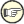#Boost C++ Libraries

...one of the most highly regarded and expertly designed C++ library projects in the world.

This is the documentation for an old version of boost. Click here for the latest Boost documentation.

## Object Interface

Basic Interface
Derived Object types
Extracting C++ objects
Enums

Python is dynamically typed, unlike C++ which is statically typed. Python variables may hold an integer, a float, list, dict, tuple, str, long etc., among other things. In the viewpoint of Boost.Python and C++, these Pythonic variables are just instances of class object. We will see in this chapter how to deal with Python objects.

As mentioned, one of the goals of Boost.Python is to provide a bidirectional mapping between C++ and Python while maintaining the Python feel. Boost.Python C++ objects are as close as possible to Python. This should minimize the learning curve significantly.### Basic Interface

Class object wraps PyObject*. All the intricacies of dealing with PyObjects such as managing reference counting are handled by the object class. C++ object interoperability is seamless. Boost.Python C++ objects can in fact be explicitly constructed from any C++ object.

To illustrate, this Python code snippet:

```def f(x, y):
if (y == 'foo'):
x[3:7] = 'bar'
else:
x.items += y(3, x)
return x

def getfunc():
return f;
```

Can be rewritten in C++ using Boost.Python facilities this way:

```object f(object x, object y) {
if (y == "foo")
x.slice(3,7) = "bar";
else
x.attr("items") += y(3, x);
return x;
}
object getfunc() {
return object(f);
}
```

Apart from cosmetic differences due to the fact that we are writing the code in C++, the look and feel should be immediately apparent to the Python coder.

### Derived Object types

Boost.Python comes with a set of derived object types corresponding to that of Python's:

• list
• dict
• tuple
• str
• long_
• enum

These derived object types act like real Python types. For instance:

```str(1) ==> "1"
```

Wherever appropriate, a particular derived object has corresponding Python type's methods. For instance, dict has a keys() method:

```d.keys()
```

make_tuple is provided for declaring tuple literals. Example:

```make_tuple(123, 'D', "Hello, World", 0.0);
```

In C++, when Boost.Python objects are used as arguments to functions, subtype matching is required. For example, when a function f, as declared below, is wrapped, it will only accept instances of Python's str type and subtypes.

```void f(str name)
{
object n2 = name.attr("upper")();   // NAME = name.upper()
str NAME = name.upper();            // better
object msg = "%s is bigger than %s" % make_tuple(NAME,name);
}
```

In finer detail:

```str NAME = name.upper();
```

Illustrates that we provide versions of the str type's methods as C++ member functions.

```object msg = "%s is bigger than %s" % make_tuple(NAME,name);
```

Demonstrates that you can write the C++ equivalent of "format" % x,y,z in Python, which is useful since there's no easy way to do that in std C++.

Python:

```>>> d = dict(x.__dict__)     # copies x.__dict__
>>> d['whatever'] = 3        # modifies the copy
```

C++:

```dict d(x.attr("__dict__"));  // copies x.__dict__
d['whatever'] = 3;           // modifies the copy
```

## class_<T> as objects

Due to the dynamic nature of Boost.Python objects, any class_<T> may also be one of these types! The following code snippet wraps the class (type) object.

We can use this to create wrapped instances. Example:

```object vec345 = (
class_<Vec2>("Vec2", init<double, double>())
)(3.0, 4.0);

assert(vec345.attr("length") == 5.0);
```

### Extracting C++ objects

At some point, we will need to get C++ values out of object instances. This can be achieved with the extract<T> function. Consider the following:

```double x = o.attr("length"); // compile error
```

In the code above, we got a compiler error because Boost.Python object can't be implicitly converted to doubles. Instead, what we wanted to do above can be achieved by writing:

```double l = extract<double>(o.attr("length"));
Vec2& v = extract<Vec2&>(o);
assert(l == v.length());
```

The first line attempts to extract the "length" attribute of the Boost.Python object. The second line attempts to extract the Vec2 object from held by the Boost.Python object.

Take note that we said "attempt to" above. What if the Boost.Python object does not really hold a Vec2 type? This is certainly a possibility considering the dynamic nature of Python objects. To be on the safe side, if the C++ type can't be extracted, an appropriate exception is thrown. To avoid an exception, we need to test for extractibility:

```extract<Vec2&> x(o);
if (x.check()) {
Vec2& v = x(); ...
```The astute reader might have noticed that the extract<T> facility in fact solves the mutable copying problem:

```dict d = extract<dict>(x.attr("__dict__"));
d["whatever"] = 3;          // modifies x.__dict__ !
```

### Enums

Boost.Python has a nifty facility to capture and wrap C++ enums. While Python has no enum type, we'll often want to expose our C++ enums to Python as an int. Boost.Python's enum facility makes this easy while taking care of the proper conversions from Python's dynamic typing to C++'s strong static typing (in C++, ints cannot be implicitly converted to enums). To illustrate, given a C++ enum:

```enum choice { red, blue };
```

the construct:

```enum_<choice>("choice")
.value("red", red)
.value("blue", blue)
;
```

can be used to expose to Python. The new enum type is created in the current scope(), which is usually the current module. The snippet above creates a Python class derived from Python's int type which is associated with the C++ type passed as its first parameter.

Notewhat is a scope? The scope is a class that has an associated global Python object which controls the Python namespace in which new extension classes and wrapped functions will be defined as attributes. Details can be found here.

You can access those values in Python as

```>>> my_module.choice.red
my_module.choice.red
```

where my_module is the module where the enum is declared. You can also create a new scope around a class:

```scope in_X = class_<X>("X")
.def( ... )
.def( ... )
;

// Expose X::nested as X.nested
enum_<X::nested>("nested")
.value("red", red)
.value("blue", blue)
;
```